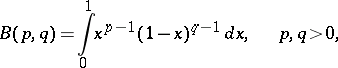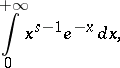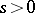Euler integrals

(diff) ← Older revision | Latest revision (diff) | Newer revision → (diff)

The integralcalled the Euler integral of the first kind, or the beta-function, andcalled the Euler integral of the second kind. (The latter converges forand is a representation of the gamma-function.)

These integrals were considered by L. Euler (1729–1731).

How to Cite This Entry:
Euler integrals. Encyclopedia of Mathematics. URL: http://www.encyclopediaofmath.org/index.php?title=Euler_integrals&oldid=16378
This article was adapted from an original article by L.D. Kudryavtsev (originator), which appeared in Encyclopedia of Mathematics - ISBN 1402006098. See original article